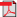# Search Results

1. R. Boucherie and P. Lassila, Efficient estimation of blocking probabilities in non-stationary loss networks, in Proceedings of 19th International Teletraffic Congress (ITC-19), pp. 1707-1716, 2005, Beijing, China(bib)
Abstract: We consider estimation of blocking probabilities in a nonstationary loss network. By invoking the so called MOL (Modified Offered Load) approximation, the problem is transformed into one requiring the solution of blocking probabilities in stationary loss networks with time varying loads. To estimate these blocking probabilities, Monte Carlo simulation is used and to increase the efficiency of the simulation, we develop a likelihood ratio method that enables samples drawn at one time point to be used at later time points. This reduces the need to draw new independent samples at every time point, thus giving substantial savings in the computational effort. The accuracy of the method is analyzed by using Taylor series approximations of the variance indicating the direct dependence of the accuracy on the rate of change of the actual load. Finally, two practical applications are provided to demonstrate the efficiency of the method.